# GED Math : Solving by Other Methods

## Example Questions

2 Next →

### Example Question #11 : Solving By Other Methods

Solve the following by using the Quadratic Formula: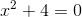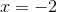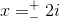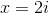No solutionExplanation: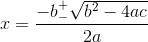Plugging into the Quadratic Formula, we get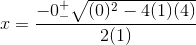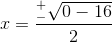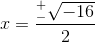*The square root of a negative number will involve the use of complex numbers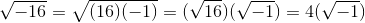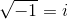Therefore,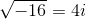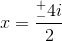### Example Question #401 : Algebra

A rectangular yard has a width of w and a length two more than three times the width. The area of the yard is 120 square feet. Find the length of the yard.

6 feet

24 feet

89 feet

20 feet

5 feet

20 feet

Explanation:

The area of the garden is 120 square feet. The width is given by w, and the length is 2 more than 3 times the width. Going by the order of operations implied, we have length given by 3w+2.

(length) x (width) = area (for a rectangle)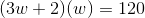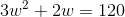In order to solve for w, we need to set the equation equal to 0.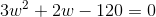To solve this we should use the Quadratic Formula: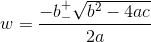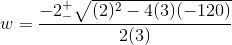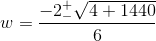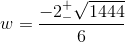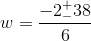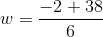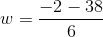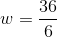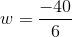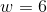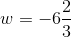(reject)

The width is 6 feet, so the length is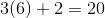or 20 feet.

### Example Question #93 : Quadratic Equations

Complete the square to solve forin the equation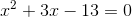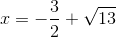or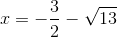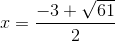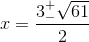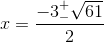Explanation:

1) Get all of the variables on one side and the constants on the other.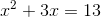2) Get a perfect square trinomial on the left side. One-half the x-term, which will be squared. Add squared term to both sides.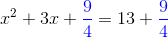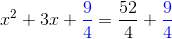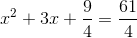3) We have a perfect square trinomial on the left side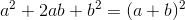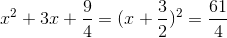4)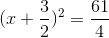5)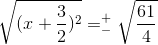6)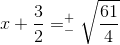7)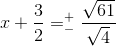8)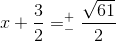9)### Example Question #94 : Quadratic Equations

Solve the following quadratic equation for x by completing the square: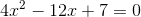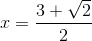or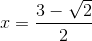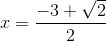or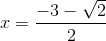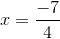or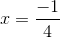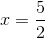orExplanation:

This quadratic equation needs to be solved by completing the square.

1) Get all of the x-terms on the left side, and the constants on the right side.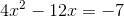2) To put this equation into terms are more common with completing the square, we can make a coefficient of 1 in front of theterm.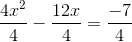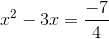3) We need to make the left side of the equation into a "perfect square trinomial." To do this, we take one-half of the coefficient in front of the x, square it, and add it to both sides.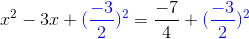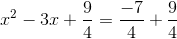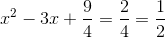The left side is a perfect square trinomial.

4) We can represent a perfect square trinomial as a binomial squared.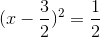5) Take the square root of both sides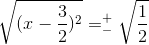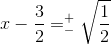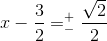6) Solve for x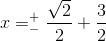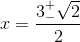2 Next →

### All GED Math Resources